# Python: Getting Started with Data Analysis¶

Analysis with Programming have recently been syndicated to Planet Python. And as a first post being a contributing blog on the said site, I would like to share how to get started with data analysis on Python. Specifically, I would like to do the following:

1. Importing the data
• Importing CSV file both locally and from the web;
2. Data transformation;
3. Descriptive statistics of the data;
4. Hypothesis testing
• One-sample t test;
5. Visualization; and
6. Creating custom function.
In :
# Import the pandas for manipulating the data
import pandas as pd

# Import the module for statistical inference
from scipy import stats as ss

# Import the module for plotting
import matplotlib.pyplot as plt

# Import the seaborn library
import seaborn as sns

# Import the numpy library
import numpy as np

# Allow ipython to draw the plots embeded in the page:
%pylab inline

Populating the interactive namespace from numpy and matplotlib

/usr/local/lib/python2.7/dist-packages/pandas/computation/expressions.py:21: UserWarning: The installed version of numexpr 1.4.2 is not supported in pandas and will be not be used
The minimum supported version is 2.1

"version is 2.1\n".format(ver=ver), UserWarning)


## Importing the data¶

This is the crucial step, we need to import the data in order to proceed with the succeeding analysis. And often times data are in CSV format, if not, at least can be converted to CSV format. In Python we can do this using the following codes:

In :
# Reading data locally

data_url = "https://raw.githubusercontent.com/alstat/Analysis-with-Programming/master/2014/Python/Numerical-Descriptions-of-the-Data/data.csv"


To read CSV file locally, we need the pandas module which is a python data analysis library.

The read_csv function can read data both locally and from the web.

## Data transformation¶

Now that we have the data in the workspace, next is to do transformation. Statisticians and scientists often do this step to remove unnecessary data not included in the analysis. Let's view the data first:

In :
# Head of the data

    Abra  Apayao  Benguet  Ifugao  Kalinga
0   1243    2934      148    3300    10553
1   4158    9235     4287    8063    35257
2   1787    1922     1955    1074     4544
3  17152   14501     3536   19607    31687
4   1266    2385     2530    3315     8520

In :
# Tail of the data
print df.tail()

     Abra  Apayao  Benguet  Ifugao  Kalinga
74   2505   20878     3519   19737    16513
75  60303   40065     7062   19422    61808
76   6311    6756     3561   15910    23349
77  13345   38902     2583   11096    68663
78   2623   18264     3745   16787    16900


To R programmers, above is the equivalent of print(head(df)) which prints the first six rows of the data, and print(tail(df)) -- the last six rows of the data, respectively. In Python, however, the number of rows for head of the data by default is 5 unlike in R, which is 6. So that the equivalent of the R code head(df, n = 10) in Python, is df.head(n = 10). Same goes for the tail of the data.

Column and row names of the data are extracted using the colnames and rownames functions in R, respectively. In Python, we extract it using the columns and index attributes. That is,

In :
# Extracting column names
print df.columns

Index([u'Abra', u'Apayao', u'Benguet', u'Ifugao', u'Kalinga'], dtype='object')

In :
# Extracting row names or the index
print df.index

Int64Index([0, 1, 2, 3, 4, 5, 6, 7, 8, 9, 10, 11, 12, 13, 14, 15, 16, 17, 18, 19, 20, 21, 22, 23, 24, 25, 26, 27, 28, 29, 30, 31, 32, 33, 34, 35, 36, 37, 38, 39, 40, 41, 42, 43, 44, 45, 46, 47, 48, 49, 50, 51, 52, 53, 54, 55, 56, 57, 58, 59, 60, 61, 62, 63, 64, 65, 66, 67, 68, 69, 70, 71, 72, 73, 74, 75, 76, 77, 78], dtype='int64')


Transposing the data is obtain using the T method,

In :
# Transpose data
print df.T

            0      1     2      3     4      5     6      7     8      9   \
Abra      1243   4158  1787  17152  1266   5576   927  21540  1039   5424
Apayao    2934   9235  1922  14501  2385   7452  1099  17038  1382  10588
Benguet    148   4287  1955   3536  2530    771  2796   2463  2592   1064
Ifugao    3300   8063  1074  19607  3315  13134  5134  14226  6842  13828
Kalinga  10553  35257  4544  31687  8520  28252  3106  36238  4973  40140

...       69     70     71     72     73     74     75     76     77  \
Abra     ...    12763   2470  59094   6209  13316   2505  60303   6311  13345
Apayao   ...    37625  19532  35126   6335  38613  20878  40065   6756  38902
Benguet  ...     2354   4045   5987   3530   2585   3519   7062   3561   2583
Ifugao   ...     9838  17125  18940  15560   7746  19737  19422  15910  11096
Kalinga  ...    65782  15279  52437  24385  66148  16513  61808  23349  68663

78
Abra      2623
Apayao   18264
Benguet   3745
Ifugao   16787
Kalinga  16900

[5 rows x 79 columns]


Other transformations such as sort can be done using sort attribute. Now let's extract a specific column. In Python, we do it using either iloc or ix attributes, but ix is more robust and thus I prefer it. Assuming we want the head of the first column of the data, we have

In :
print df.ix[:, 0].head()

0     1243
1     4158
2     1787
3    17152
4     1266
Name: Abra, dtype: int64


By the way, the indexing in Python starts with 0 and not 1. To slice the first three columns of the 10th to 20th rows, run the following

In :
print df.ix[10:20, 0:3]

     Abra  Apayao  Benguet
10    981    1311     2560
11  27366   15093     3039
12   1100    1701     2382
13   7212   11001     1088
14   1048    1427     2847
15  25679   15661     2942
16   1055    2191     2119
17   5437    6461      734
18   1029    1183     2302
19  23710   12222     2598
20   1091    2343     2654


Which is equivalent to print df.ix[10:20, ['Abra', 'Apayao', 'Benguet']]

To drop a column in the data, say columns 1 (Apayao) and 2 (Benguet), use the drop attribute. That is,

In :
print df.drop(df.columns[[1, 2]], axis = 1).head()

    Abra  Ifugao  Kalinga
0   1243    3300    10553
1   4158    8063    35257
2   1787    1074     4544
3  17152   19607    31687
4   1266    3315     8520


axis argument above tells the function to drop with respect to columns, if axis = 0, then the function drops with respect to rows.

## Descriptive Statistics¶

Next step is to do descriptive statistics for preliminary analysis of our data using the describe attribute:

In :
print df.describe()

               Abra        Apayao      Benguet        Ifugao       Kalinga
count     79.000000     79.000000    79.000000     79.000000     79.000000
mean   12874.379747  16860.645570  3237.392405  12414.620253  30446.417722
std    16746.466945  15448.153794  1588.536429   5034.282019  22245.707692
min      927.000000    401.000000   148.000000   1074.000000   2346.000000
25%     1524.000000   3435.500000  2328.000000   8205.000000   8601.500000
50%     5790.000000  10588.000000  3202.000000  13044.000000  24494.000000
75%    13330.500000  33289.000000  3918.500000  16099.500000  52510.500000
max    60303.000000  54625.000000  8813.000000  21031.000000  68663.000000


## Hypothesis Testing¶

Python has a great package for statistical inference. And that's the stats library of scipy. The one sample t-test is implemented in ttest_1samp function. So that, if we want to test the mean of the Abra's volume of palay production against the null hypothesis with 1500 assumed population mean of the volume of palay production, we have

In :
# Perform one sample t-test using 1500 as the true mean
print ss.ttest_1samp(a = df.ix[:, 'Abra'], popmean = 15000)

(-1.1281738488299586, 0.26270472069109496)


The values returned are tuple of the following values:

• t : float or array
• t-statistic
• prob : float or array
• two-tailed p-value

From the above numerical output, we see that the p-value = 0.2627 is greater than α=0.05, hence there is no sufficient evidence to conclude that the average volume of palay production is not equal to 15000. Applying this test for all variables against the population mean 15000 volume of production, we have

In :
print ss.ttest_1samp(a = df, popmean = 15000)

(array([ -1.12817385,   1.07053437, -65.81425599,  -4.564575  ,   6.17156198]), array([  2.62704721e-01,   2.87680340e-01,   4.15643528e-70,
1.83764399e-05,   2.82461897e-08]))


The first array returned is the t-statistic of the data, and the second array is the corresponding p-values.

## Visualization¶

There are several module for visualization in Python, and the most popular one is the matplotlib library. To mention few, we have bokeh and seaborn modules as well to choose from. In my previous post, I've demonstrated the matplotlib package which has the following graphic for box-whisker plot,

In :
# added to allow to see the result with the basic matplotlib config even when running the code again, (as the next cell command
# overwrite the global matplolib config)
matplotlib.rcdefaults()

plt.show(df.plot(kind = 'box'))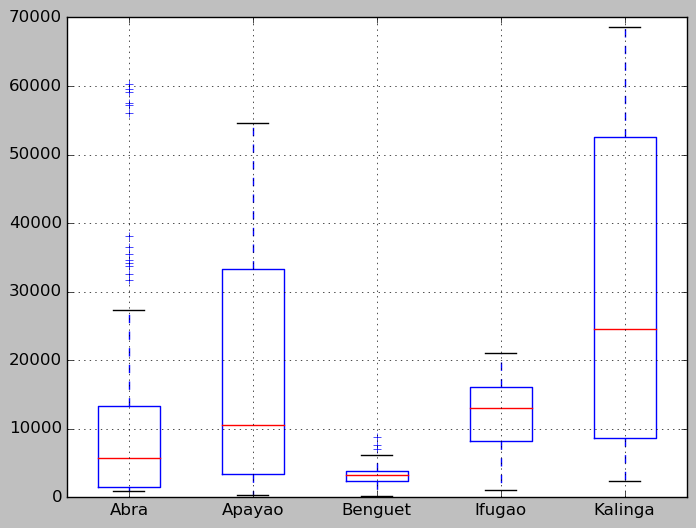Now plotting using pandas module can beautify the above plot into the theme of the popular R plotting package, the ggplot. To use the ggplot theme just add one more line to the above code,

In :
pd.options.display.mpl_style = 'default' # Sets the plotting display theme to ggplot2


And you'll have the following,

In :
df.plot(kind = 'box')

Out:
<matplotlib.axes._subplots.AxesSubplot at 0x56eb690>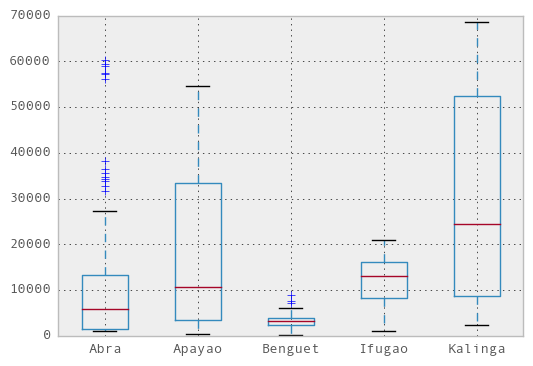In :
# Do the boxplot
plt.show(sns.boxplot(df, widths = 0.5, color = "pastel"))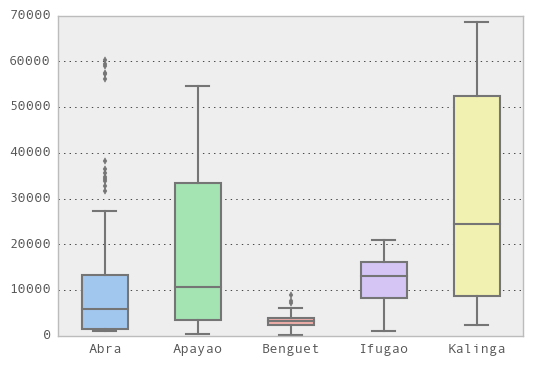Even neater than the default matplotlib.pyplot theme. But in this post, I would like to introduce the seaborn module which is a statistical data visualization library. So that, we have the following

In :
plt.show(sns.violinplot(df, widths = 0.5, color = "pastel"))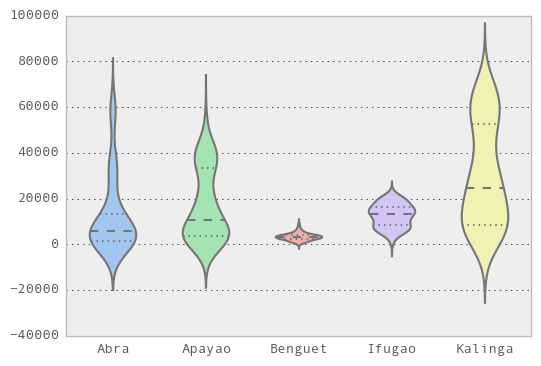Sexy boxplot, scroll down for more.

In :
plt.show(sns.distplot(df.ix[:,2], rug = True, bins = 15))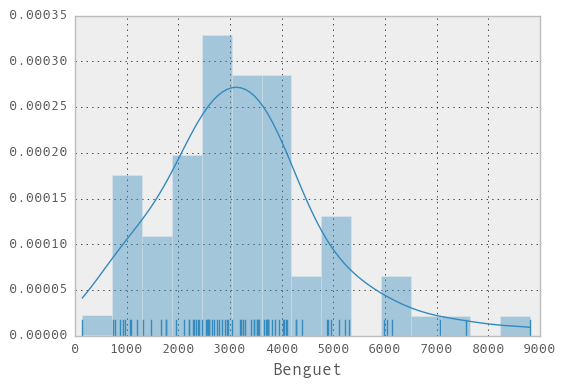In :
with sns.axes_style("white"):
plt.show(sns.jointplot(df.ix[:,1], df.ix[:,2], kind = "kde"))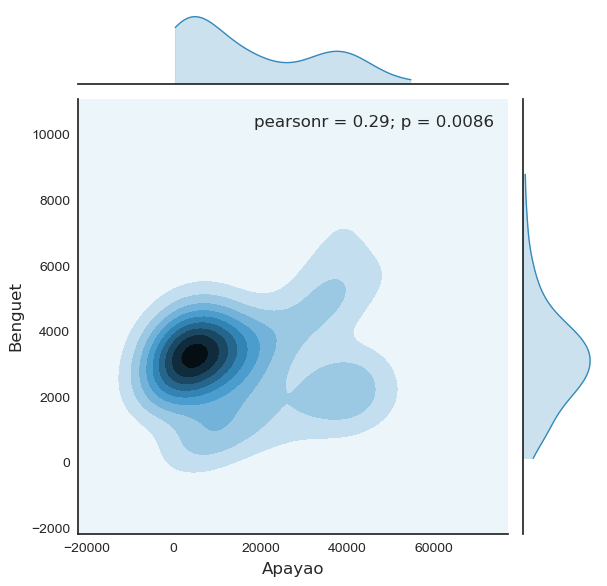In :
plt.show(sns.lmplot("Benguet", "Ifugao", df))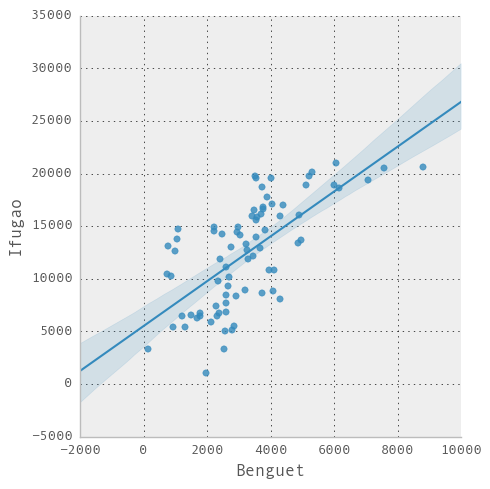## Creating custom function¶

To define a custom function in Python, we use the def function. For example, say we define a function that will add two numbers, we do it as follows,

In :
def add_2int(x, y):
return x + y


4


By the way, in Python indentation is important. Use indentation for scope of the function, which in R we do it with braces {...}. Now here's an algorithm from my previous post,

1. Generate samples of size 10 from Normal distribution with $μ = 3$ and $σ^2 = 5$;
2. Compute the $\bar{x}$ and $\bar{x}∓z_{\alpha/2}\frac{σ}{\sqrt{n}}$ using the 95% confidence level;
3. Repeat the process 100 times; then
4. Compute the percentage of the confidence intervals containing the true mean.

Coding this in Python we have,

In :
def case(n = 10, mu = 3, sigma = np.sqrt(5), p = 0.025, rep = 100):
m = np.zeros((rep, 4))

for i in range(rep):
norm = np.random.normal(loc = mu, scale = sigma, size = n)
xbar = np.mean(norm)
low = xbar - ss.norm.ppf(q = 1 - p) * (sigma / np.sqrt(n))
up = xbar + ss.norm.ppf(q = 1 - p) * (sigma / np.sqrt(n))

if (mu > low) & (mu < up):
rem = 1
else:
rem = 0

m[i, :] = [xbar, low, up, rem]

inside = np.sum(m[:, 3])
per = inside / rep
desc = "There are " + str(inside) + " confidence intervals that contain " \
"the true mean (" + str(mu) + "), that is " + str(per) + " percent of the total CIs"

return {"Matrix": m, "Decision": desc}


Above code might be easy to read, but it's slow in replication. Below is the improvement of the above code, thanks to Python gurus, see 15 Comments on my previous post.

In :
def case2(n = 10, mu = 3, sigma = np.sqrt(5), p = 0.025, rep = 100):
scaled_crit = ss.norm.ppf(q = 1 - p) * (sigma / np.sqrt(n))
norm = np.random.normal(loc = mu, scale = sigma, size = (rep, n))

xbar = norm.mean(1)
low = xbar - scaled_crit
up = xbar + scaled_crit

rem = (mu > low) & (mu < up)
m = np.c_[xbar, low, up, rem]

inside = np.sum(m[:, 3])
per = inside / rep
desc = "There are " + str(inside) + " confidence intervals that contain " \
"the true mean (" + str(mu) + "), that is " + str(per) + " percent of the total CIs"
return {"Matrix": m, "Decision": desc}


## Data Source¶

Philippine Bureau of Agricultural Statistics

## Reference¶

1. Pandas, Scipy, and Seaborn Documentations.
2. Wes McKinney & PyData Development Team (2014). pandas: powerful Python data analysis toolkit.